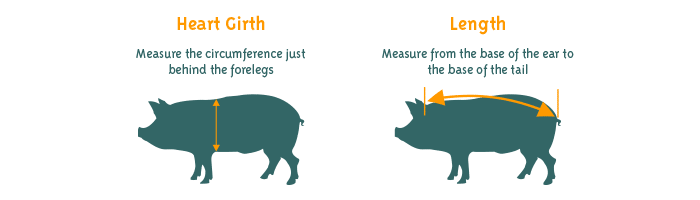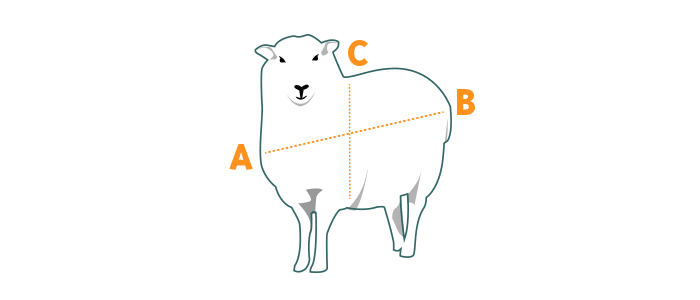01582 842096 01582 842096

Open until 6:00pm
• Own Brand
• Frozen & Raw Food

# Farm Animal Health Products

## How heavy is my Pig? How heavy is my Sheep/Goat?

For most types of medication, it is important to know the weight of the animal to be treated. Ideally some sort of weighing scale is used, but failing that it is much better to calculate this from measurements taken off the animal than to guess. The information below can be used to help with this:

### To find the weight of a Pig1. Use the same procedure as above taking your measurement in Meters.
Then use the following formula.
2. Square the Heart Girth to get the Girth Result.
3. Now Multiply the Girth Result by the Length and MULTIPLY by 69.3
4. You now have the weight of your pig in Kg.

Example:

• Porky Pig has a Heart Girth of 1.27 meters and a Length of 1.02 meters.
• Squaring the Heart Girth (1.27 x 1.27) = 1.6129 = Girth Result
• Multiply the Girth Result (1.6129) by the Length (1.02) and MULTIPLY by 69.3 = 114 Kg.

### To find the weight of a Sheep or Goat1. Measure the circumference of the animal, as shown in distance C in the illustration. Make sure to measure girth in relation to the location of the animal's heart. On a sheep, ensure an accurate measurement by compressing the sheep's wool so that the circumference reflects that of the body and does not include that of the body plus the wool.
2. Measure the length of the animal's body, as shown in distance A-B in the illustration.
3. Using the measurements from steps 1 and 2, calculate body weight using the formula HEART GIRTH x HEART GIRTH x BODY LENGTH / 300 = ANIMAL WEIGHT IN POUNDS. For example, if a sheep has a heart girth equal to 35 inches and a body length equal to 30 inches, the calculation would be (35 x 35 x 30) / 300 = 122 lbs.